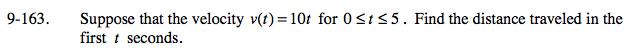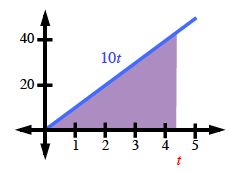### Home > PC > Chapter 9 > Lesson 9.3.5 > Problem9-163

9-163.

Suppose that the velocity v(t) = 10t for 0 ≤ t ≤ 5. Find the distance traveled in the first t seconds. Homework Help ✎Find the area under the curve from 0 to t.

$\text{area}=\frac{(\text{base})(\text{height})}{2}$